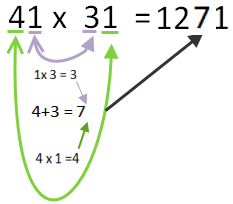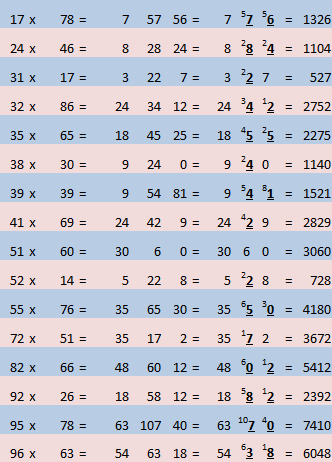Fast Multiplication of 2 digit numbers under 100

Imagine when you are asked to multiply two numbers that none of the previous tricks  (eg close to 100, between 11-19) can be used.

A  fast method to multiply 2 digit by 2 digit numbers.
This is known as Rainbow Multiplication.

Let us use 41×31 for example.
41 x 31

1)    Multiply the 1st digit of the 1st number (4) by the 2nd digit of the 2nd number (3) to get the 1st digit(s) of your answer (it will be 2 digits if it is greater than 9)  .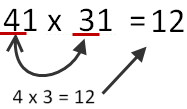2)    Put a blank space after the 1st digit(s) of the answer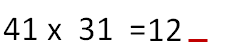3)    Multiply the 2nd  digit of the 1st number (1) by the 2nd digit of the 2nd number (1) to get the last digit(s) of your answer.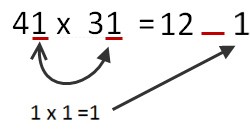4)    To find what the blank space is find the product of the outside digits (4×1=12) and add to it the product of the inside digits ( 1×3 =3) .  4+3=7.